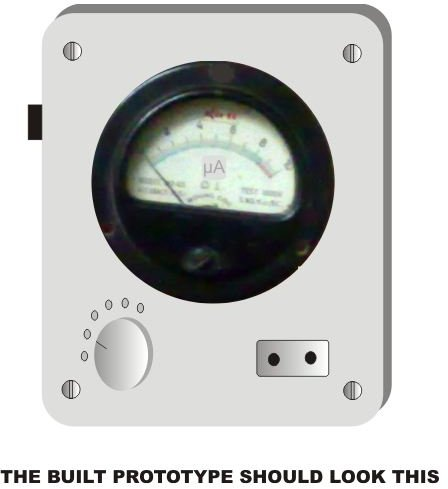# How to Build a Simple Analogue Capacitance Meter

Page content

## Introduction

The different models of digital multimeters available in the market are all equipped to measure most electronic quantities. But surprisingly, almost all of these don’t include a capacitor measuring facility. The meters that have this may be too expensive to procure for new electronic enthusiasts and engineers. The circuit design of a simple capacitance meter presented here is analogue in operation, yet, can definitely prove to be a very useful tool for the measurements of capacitors with reasonably good variable ranges.

The operating concept is pretty basic. The square waves produced by a CMOS oscillator IC are used to alternately charge and discharge the unknown capacitor. The average current required for the procedure is directly displayed over an analogue moving coil type of meter. The current, being directly proportional to the charging rate of the capacitor, always produces an equalvalent reading on the meter (after proper calibration) and thus can be read directly.

## Circuit Description

Refer to the figure so the circuit functioning may be understood with the given points:

(Click Image to Enlarge)

• CMOS IC 4049, which contains 6 inverters in one pack, forms the main active part of the whole circuit.

• Two of its gates N1 and N2 are rigged as an astable multivibrator, with VR1, R1 and S1 (variable) determining the frequency of the oscillator.

• The signal at the output of N2 is invariably a square wave. The square waves are basically just continuous and alternate generation of the positive voltage and zero voltage.

• Gates N3 to N6 are all arranged as buffers to reduce load dependence of the circuit, C7 and R2 act as integrators to stabilize the meter needle pointer.

• The capacitor CX which is to be measured is connected to the output of the buffers as shown in the figure.

• During a positive peak of the square wave, the unknown capacitor CX charges up to the supply voltage and at the next zero voltage pulse it discharges almost instantly through D1.

## Parts List

All Resistors are ¼ watt, carbon film, 5% tolerance type (unless otherwise specified)

R1 = 100 K

R2 = 10 K,

VR1 = 10 K,

C1 = 470 p, C2 = 4.7 n, C3 = 0.047 µ, C4 = 0.47 µ, C8 = 104 (All Ceramic Disc),

C5 = 4.7 µ/ 25V, C6 = 47 µ/ 25V, C7, C9 = 10 µ/ 25V, C10 = 100 µ/ 25V (All Electrolytic),

D1 = 1N4148,

N1 TO N6 = IC 4049,

General PCB As Per Size,

Moving Coil Type Ammeter = 100 µA, Full Scale Deflection

## Relation Between Current and Capacitance

Before learning about how to test a capacitor, it would be interesting first to find out regarding the mathematics involved during the testing process. Let’s read the brief explanation.

The charge Q on the capacitor may be expressed using the following formula:

Q = CV, where C is the capacitance and V is the supply voltage.

Now since the current involved during the process is given as:

I = Q × F, where F is the frequency of the square wave.

Replacing Q = CV in the above formula, we get:

I = CVF, clearly indicating that the capacitance C is directly proportional to the current I, provided, the frequency is constant.

Also, since the supply voltage is 9 volts, we finally have:

I = 9CF; this magnitude of current will reach the meter and will be responsible for the relevant readings.

Thus through calculations using the above formula a micro ammeter is initially calibrated suitably for a direct reading of the capacitor values in question.

## Construction HintsWith the help of the shown schematic,the circuit is easily built through the following steps:

• Begin by inserting the IC somewhere in the middle of the general PCB and solder all its leads.

• Organize the associated components in the best possible orientation around the IC and solder their interconnections.

• Similarly finish assembling the power supply section over the PCB and do the necessary interconnections.

• Connect the associated ammeter to the assembled circuit.

• Enclose the entire assembly in a suitable plastic or wooden box, having the required cut outs for fixing the meter, capacitor sockets and the outlet for the mains cord to come out (Click Image to Enlarge)

This concludes the construction procedure; now let’s learn how to set up the unit.

## Calibration

The calibration of this capacitance meter is simply done with the following instructions:

• Take a relatively small value capacitor whose value is accurately known (for example a 0.1uF ceramic disc). Fix it in the capacitor socket provided for checking the values of the unknown capacitors.

• Switch ON the power supply - select a suitable position on S1 so that the deflection on the meter reads near about full scale. Fine tune VR1 to make the reading exactly full scale.

• Due to a linear behavior of the circuit, the readings will perfectly respond to other values of capacitors proportionately throughout the entire meter calibration.

• This effort should take care of the calibration and now your meter is ready for use.

Still wondering how to test a capacitor? Well, now it’s a matter of just inserting the unknown capacitor terminals into the given socket and getting a direct reading over the meter. Simple isn’t it? But remember to adjust the switch S1 so that the maximum range is selected appropriately.

Do not forget to discharge (by shorting leads) large capacitors before checking, otherwise the stored charge may damage your meter.